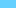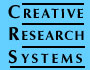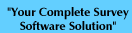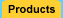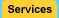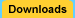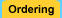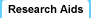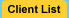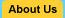Sample Size Calculator Significance Survey Design Correlation Interviewing Companies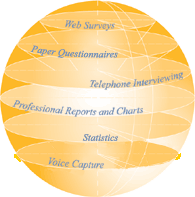# Correlation

Correlation is a statistical technique which can show whether and how strongly pairs of variables are related. For example, height and weight are related - taller people tend to be heavier than shorter people. The relationship isn't perfect. People of the same height vary in weight, and you can easily think of two people you know where the shorter one is heavier than the taller one. Nonetheless, the average weight of people 5'5'' is less than the average weight of people 5'6'', and their average weight is less than that of people 5'7'', etc. Correlation can tell you just how much of the variation in peoples' weights is related to their heights.

Although this correlation is fairly obvious your data may contain unsuspected correlations. You may also suspect there are correlations, but don't know which are the strongest. An intelligent correlation analysis can lead to a greater understanding of your data.

There are several different correlation techniques. The Survey System's optional Statistics Module includes the most common type, called the Pearson or product-moment correlation. The module also includes a variation on this type called partial correlation. The latter is useful when you want to look at the relationship between two variables while removing the effect of one or two other variables.

Like all statistical techniques, correlation is only appropriate for certain kinds of data. Correlation works for data in which numbers are meaningful, usually quantities of some sort. It cannot be used for purely categorical data, such as gender, brands purchased or favorite color.

Rating scales are a controversial middle case. The numbers in rating scales have meaning, but that meaning isn't very precise. They are not like quantities. With a quantity (such as dollars), the difference between 1 and 2 is exactly the same as between 2 and 3. With a rating scale, that isn't really the case. You can be sure that your respondents think a rating of 2 is between a rating of 1 and a rating of 3, but you cannot be sure they think it is exactly halfway between. This is especially true if you labeled the mid-points of your scale (you cannot assume "good" is exactly half way between "excellent" and "fair").

Most statisticians say you cannot use correlations with rating scales, because the mathematics of the technique assume the differences between numbers are exactly equal. Nevertheless, many survey researchers do use correlations with rating scales, because the results usually reflect the real world. Our own position is that you can use correlations with rating scales, but you should do so with care. When working with quantities, correlations provide precise measurements. When working with rating scales, correlations provide general indications.

The main result of a correlation is called the correlation coefficient (or "r"). It ranges from -1.0 to +1.0. The closer r is to +1 or -1, the more closely the two variables are related.

If r is close to 0, it means there is no relationship between the variables. If r is positive, it means that as one variable gets larger the other gets larger. If r is negative it means that as one gets larger, the other gets smaller (often called an "inverse" correlation).

While correlation coefficients are normally reported as r = (a value between -1 and +1), squaring them makes then easier to understand. The square of the coefficient (or r square) is equal to the percent of the variation in one variable that is related to the variation in the other. After squaring r, ignore the decimal point. An r of .5 means 25% of the variation is related (.5 squared =.25). An r value of .7 means 49% of the variance is related (.7 squared = .49).

A correlation report can also show a second result of each test - statistical significance. In this case, the significance level will tell you how likely it is that the correlations reported may be due to chance in the form of random sampling error. If you are working with small sample sizes, choose a report format that includes the significance level. This format also reports the sample size.

A key thing to remember when working with correlations is never to assume a correlation means that a change in one variable causes a change in another. Sales of personal computers and athletic shoes have both risen strongly in the last several years and there is a high correlation between them, but you cannot assume that buying computers causes people to buy athletic shoes (or vice versa).

The second caveat is that the Pearson correlation technique works best with linear relationships: as one variable gets larger, the other gets larger (or smaller) in direct proportion. It does not work well with curvilinear relationships (in which the relationship does not follow a straight line). An example of a curvilinear relationship is age and health care. They are related, but the relationship doesn't follow a straight line. Young children and older people both tend to use much more health care than teenagers or young adults. Multiple regression (also included in the Statistics Module) can be used to examine curvilinear relationships, but it is beyond the scope of this article.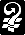Copyright © 2002  Creative Research Systems  (707) 765-1001  info@surveysystem.com